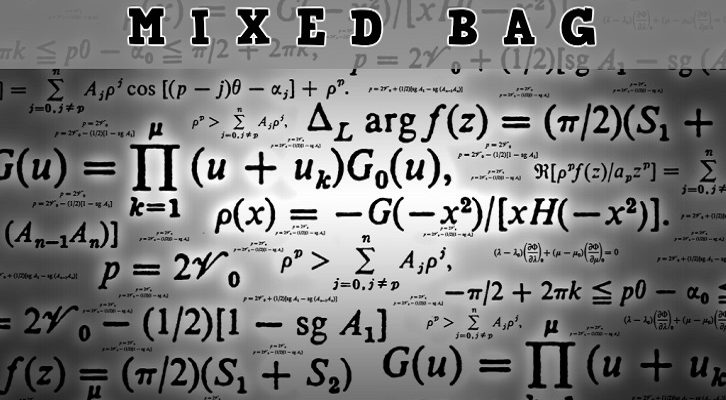# CAT Functions

Questions from functions have increased over the past few years. Given below is an interesting question from functions.

Question

Consider functions  f(x) = x2 + 2x,  g(x) = √(x +1) and h(x) = g(f(x)). What are the domain and range of h(x)?

Domain = ( – infinity, +infinity)
Range – [0, infinity]